# 5.8: Orbitals

$$\newcommand{\vecs}{\overset { \rightharpoonup} {\mathbf{#1}} }$$ $$\newcommand{\vecd}{\overset{-\!-\!\rightharpoonup}{\vphantom{a}\smash {#1}}}$$$$\newcommand{\id}{\mathrm{id}}$$ $$\newcommand{\Span}{\mathrm{span}}$$ $$\newcommand{\kernel}{\mathrm{null}\,}$$ $$\newcommand{\range}{\mathrm{range}\,}$$ $$\newcommand{\RealPart}{\mathrm{Re}}$$ $$\newcommand{\ImaginaryPart}{\mathrm{Im}}$$ $$\newcommand{\Argument}{\mathrm{Arg}}$$ $$\newcommand{\norm}{\| #1 \|}$$ $$\newcommand{\inner}{\langle #1, #2 \rangle}$$ $$\newcommand{\Span}{\mathrm{span}}$$ $$\newcommand{\id}{\mathrm{id}}$$ $$\newcommand{\Span}{\mathrm{span}}$$ $$\newcommand{\kernel}{\mathrm{null}\,}$$ $$\newcommand{\range}{\mathrm{range}\,}$$ $$\newcommand{\RealPart}{\mathrm{Re}}$$ $$\newcommand{\ImaginaryPart}{\mathrm{Im}}$$ $$\newcommand{\Argument}{\mathrm{Arg}}$$ $$\newcommand{\norm}{\| #1 \|}$$ $$\newcommand{\inner}{\langle #1, #2 \rangle}$$ $$\newcommand{\Span}{\mathrm{span}}$$$$\newcommand{\AA}{\unicode[.8,0]{x212B}}$$

A characteristic of the diagram Figure 1 in Electron Waves in the Hydrogen Atom is that it has been assigned an identifying label, namely, 1s. This enables us to distinguish it from other wave patterns the electron could possibly adopt if it moved about the nucleus with a higher energy. Each of these three-dimensional wave patterns is different in shape, size, or orientation from all the others and is called an orbital. The word orbital is used in order to make a distinction between these wave patterns and the circular or elliptical orbits of the Bohr picture shown in The Wave Nature of the Electron.

At ordinary temperatures, the electron in a hydrogen atom is almost invariably found to have the lowest energy available to it. That is, the electron occupies the 1s orbital. The electron cloud looks like the dot-density diagram shown in Figure 1 from Electron Waves in the Hydrogen Atom. This orbital is shown below as both a boundary surface diagram1 and a dot density diagram1

Table $$\PageIndex{1}$$: 1s Orbital
Orbital Dot Density Diagram(s) Boundary Surface Diagram
1sA vertical and horizontal axes is labeled "z" and "y" respectively. Most of the black dots are concentrated in the center. Only a few black dots are located away from the central dense region of black dots.A three dimensional axes is shown that are perpendicular to each other. The "x" axes is pointing towards us, the "y" axes is pointing towards the right and the "z" axes is pointing straight upwards. Located in the axis origin is a small red sphere.

At a very high temperature, though, some collisions between the atoms are sufficiently hard to provide one of the electrons with enough energy so that it can occupy one of the other orbitals, say a 2s orbital, but this is unusual. Nevertheless, a knowledge of these higher energy orbitals is necessary since electron clouds having the same shapes as hydrogen are found to apply to all the other atoms in the periodic table as well. The 2s orbital is shown below, once again represented by a dot density diagram and a boundary surface diagram. Notice how the dot density diagram reveals a feature about the 2s orbital that boundary surface does not: A node divides the 2s orbital in two, a portion of the electron cloud is near the center, while another portion lies beyond the node (the circular region with no dots). At the node the wave has no amplitude, its square is also zero, and there is zero probability of finding the electron.

Table $$\PageIndex{2}$$: 2s Orbital

2s Orbital
Orbital Dot Density Diagram(s) Boundary Surface Diagram
2sA vertical and horizontal axes is labeled "z" and "y" respectively. Right in the origin is a circular region where there is a large number of black dots. Beyond this center region is a ring shaped gap where there are very few to no dots. Beyond the gap is a ring shaped region concentrated with black dots.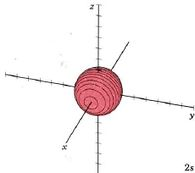A three dimensional axes is shown that are perpendicular to each other. The "x" axes is pointing towards us, the "y" axes is pointing towards the right and the "z" axes is pointing straight upwards. Right in the origin is a red sphere.

In the case of a particle in a one-dimensional box, the energy was determined by a positive whole number n. Much the same situation prevails in the case of the hydrogen atom. An integer called the principal quantum number, also designated by the symbol n, is used to label each orbital. The larger the value of n, the greater the energy of the electron and the larger the average distance of the electron cloud from the nucleus. In the two orbitals already considered, n = 1 for the 1s orbital, n= 2 for the 2s orbital.

Because a greater number of different shapes is available in the case of three-dimensional, as opposed to one-dimensional, waves, two other labels are used in addition to n. The first consists of one of the lowercase letters s, p, d, or f. These tell us about the overall shapes of the orbitals.2 Thus all s orbitals such as the 1s, 2s are spherical. An important point is that only a limited number of orbital shapes is possible for each value of n. If n = 1, then only the spherical 1s orbital is possible. When n is increased to 2, though, two orbital types (2s and 2p) become possible. Thus along with the 2s orbital, 3 other orbitals exist when n=2; 2px, 2py, and 2pz. All p orbitals have a dumbbell shape. The third kind of label are subscripts which distinguish between orbitals which are basically the same shape but differ in their orientation in space. In the case of p orbitals there are always three orientations possible. A p orbital which extends along the x axis is labeled a px orbital. A p orbital along the y axis is labeled py and one along the z axis is a pz orbital. Below are dot density diagrams, boundary surface diagrams, and a rotating image. Using the moving images, it is easy to see that the only difference between the 2p orbitals is their orientation in xyz 3-coordinate space.

Table $$\PageIndex{3}$$: 2p Orbitals
Orbital Dot Density Diagram(s) Boundary Surface Diagram Rotating Image
2px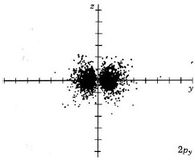A vertical and horizontal axes is labeled "z" and "y" respectively. The two lobe shaped region is concentrated with black dots and is located on opposite sides of the "y" axes. There are less and less black dots as we move further away from the center of the two axes.A three dimensional axes is shown that are perpendicular to each other. The "x" axes is pointing towards us, the "y" axes is pointing towards the right and the "z" axes is pointing straight upwards. Two red spheres located on opposite sides of the "x" axes meet at the origin.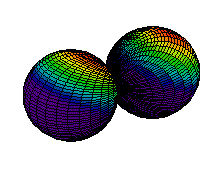Gif of two horizontally rotating spheres, each with a continuous spectrum of colors from deep violet to red, corresponding to the different probability regions of finding electrons. Red regions are concentrated near the top end of both spheres. Concentric regions around from this end change colors from red to yellow, green, blue and finally purple. The bottom half of both spheres are occupied by purple regions.
2pyA vertical and horizontal axes is labeled "z" and "y" respectively. The two lobe shaped region is concentrated with black dots and is located on opposite sides of the "y" axes. There are less and less black dots as we move further away from the center of the two axes.A three dimensional axes is shown that are perpendicular to each other. The "x" axes is pointing towards us, the "y" axes is pointing towards the right and the "z" axes is pointing straight upwards. Two red spheres located on opposite sides of the "y" axes meet at the origin.Gif of two horizontally rotating spheres, each with a continuous spectrum of colors from deep violet to red, corresponding to the different probability regions of finding electrons. Red regions are concentrated near the top end of both spheres. Concentric regions around from this end change colors from red to yellow, green, blue and finally purple. The bottom half of both spheres are occupied by purple regions.
2pzA vertical and horizontal axes is labeled "z" and "y" respectively. The two lobed shape region is concentrated with black dots and is located on opposite sides of the "z" axes. There are less and less black dots as we move further away from the center of the two axes.A three dimensional axes is shown that are perpendicular to each other. The "x" axes is pointing towards us, the "y" axes is pointing towards the right and the "z" axes is pointing straight upwards. Two red spheres located on opposite sides of the "z" axes meet at the origin.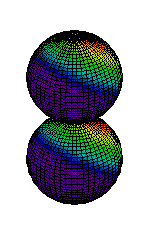Gif of two spheres stacked vertically, rotating in unison. Each sphere has a continuous spectrum of colors from deep violet to red, corresponding to the different probability regions of finding electrons. Red regions are concentrated near the top end of both spheres. Concentric regions around from this end change colors from red to yellow, green, blue and finally purple. The bottom half of both spheres are occupied by purple regions.

When n equals 3, three orbital types occur. The first two are familiar, the s orbital and p orbitals. The third, the d orbital, is discussed later. Below are representations of the 3s orbital, and the 3p orbitals. As the 2s orbital was slightly different in shape from the 1s orbital due to the introduction of a node, so the 3s and 3p orbitals differ slightly in shape from the 2s and 2p orbitals.

Table $$\PageIndex{4}$$: 3s and 3p orbitals
Orbital Dot Density Diagram(s)

Boundary Surface Diagram

3sA vertical and horizontal axes is labeled "z" and "y" respectively. The circular area around the origin is concentrated with black dots. The two other regions with concentrated black dots form concentric circles with the origin. The spaces between each concentrated region has very few to no black dots in it. Fewer and fewer black dot are present as we move further away from the center.A three dimensional axis is shown that are perpendicular to each other. The "x" axis is pointing towards us, the "y" axis is pointing towards the right and the "z" axis is pointing straight upwards. A relatively large sphere occupies a large portion of the axes. The sphere has the center at the axis origin.
3pxA vertical and horizontal axes is labeled "z" and "x" respectively. There is a relatively small circular region around the axis origin on opposites sides of the "x" axes. The other two regions are quarter annulus shaped and is symmetrical about both axes. There is a gap between the central regions and the outer region where very few to no black dots are present. The central region is more concentrated than the outer region.A three dimensional axes is shown that are perpendicular to each other. The "x" axes is pointing towards us, the "y" axes is pointing towards the right and the "z" axes is pointing straight upwards. The view from this angle shows that there are two relatively large red spheres located on opposite sides of the "x" axes.
3pyA vertical and horizontal axes is labeled "z" and "y" respectively. There is a relatively small circular concentrated region around the axis origin on opposites sides of the "y" axes. The other two regions are quarter annulus shaped and is symmetrical about both axes. There is a gap between the central regions and the outer region where very few to no black dots are present. The central region is far more concentrated than the outer region.A three dimensional axes is shown that are perpendicular to each other. The "x" axes is pointing towards us, the "y" axes is pointing towards the right and the "z" axes is pointing straight upwards. There are two small spheres around the origin on opposite sides of the "y" axes. Following this region is a small gap followed by another sphere. The side of the sphere facing the central region is dented. The two spheres located on the "y" axes are symmetrical about all axes.
3pzA vertical and horizontal axes is labeled "z" and "y" respectively. There is a relatively small circular concentrated region around the axis origin on opposites sides of the "z" axes. The other two regions are quarter annulus shaped and is symmetrical about both axes. There is a gap between the central regions and the outer region where very few to no black dots are present. The central region is far more concentrated than the outer region.A three dimensional axes is shown that are perpendicular to each other. The "x" axes is pointing towards us, the "y" axes is pointing towards the right and the "z" axes is pointing straight upwards. There are two small spheres around the origin on opposite sides of the "z" axes. Following this region is a small gap followed by another sphere. The side of the sphere facing the central region is dented. The two spheres located on the "z" axes are symmetrical about all axes.

The d orbitals have more complex shapes than the p orbitals, In the case of the d orbitals the subscripts are more difficult to follow. You can puzzle them out from the rotating images, the dot density diagrams and the orbital surface diagrams if you like, but analysis of these orbitals is usually considered beyond the scope of general chemistry. You should, however, be aware that there are five possible orientations for d orbitals. Below are representations of the d orbitals.

Table $$\PageIndex{5}$$: 3d Orbitals
Orbital Dot Density Diagram(s) Boundary Surface Diagram Rotating Image
3dxyA vertical and horizontal axes is labeled "x" and "y" respectively. There is a lobe shaped region of concentrated black dots in each quadrant of the axis which collectively makes an "X" shaped area centralized on the axis origin. There are fewer and fewer black dots as we move away from the axis origin.A three dimensional axes is shown that are perpendicular to each other. The "x" axes is pointing towards us, the "y" axes is pointing towards the right and the "z" axes is pointing straight upwards. There are four three-dimensional structures that are located on each quadrant of the "x" "y" plane. The structures are lobe shaped and form a "X" shape about the origin.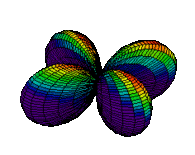Gif of a three dimensional structure rotating about the vertical "z" axes. The structure lies on the "x" and "y" plane and consist of four lobes forming a "X" shape centralized at the origin. The top half surface of the four lobes make up a continuous spectrum of colors from red to yellow to green to blue and to purple. The purple regions make up the bottom half of the lobes and makes a major portion of the structure.
3dxzA vertical and horizontal axes is labeled "z" and "x" respectively. There is a lobe shaped region of concentrated black dots in each quadrant of the axis which collectively makes an "X" shaped area centralized on the axis origin. There are fewer and fewer black dots as we move away from the axis origin.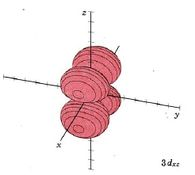A three dimensional axes is shown that are perpendicular to each other. The "x" axes is pointing towards us, the "y" axes is pointing towards the right and the "z" axes is pointing straight upwards. There are four three-dimensional structures that are located on each quadrant of the "x" "z" plane. The structures are lobe shaped and form a "X" shape about the origin.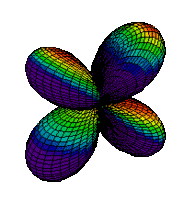Gif of a three dimensional structure rotating about the vertical "z" axes. The structure lies on the "x" and "z" plane and consist of four lobes forming a "X" shape centralized at the origin. The surface of the lobes have a continuous spectrum of color from red to yellow to green, blue and purple. The spectrum of color moves around the surface of each lobe according to its rotating position. The red, yellow, green and blue region moves around the surface of the lobes to always accumulate on the right side of the structure. The purple region make up the rest of the structure as it rotates.
3dz2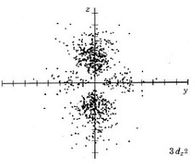A vertical and horizontal axes is labeled "z" and "y" respectively. There is a symmetrical pair of lobe shaped black dot concentrated reigons on opposite sides of the "z" axes. There is another symmetrical pair of lobe shaped black dot concentrated regions on opposite sides of the "y" axis. Note that the latter region is significantly smaller in size than the former one. Note that there is a gap around the origin where very few to no dots are present.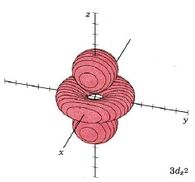A three dimensional axes is shown that are perpendicular to each other. The "x" axes is pointing towards us, the "y" axes is pointing towards the right and the "z" axes is pointing straight upwards. The two lobe shaped three dimensional structures are symmetrical and are on opposite sides of the "z" axis. Between these two lobes, on the "x" "y" plane, is a three dimensional ring centralized on the axis origin.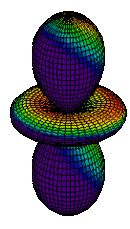Gif of a three dimensional structure rotating about the vertical "z" axes. The structure consists of two identical three dimensional lobes located on opposite sides of the "z" axis. Between the two lobes is a three dimensional ring located on the "x" "y" plane. The entire structure is rotating about the "z" axis. The surface of the lobes and the ring have a continuous spectrum of color from red to yellow to green, blue and purple. The red regions are concentrated on the right sided face of the structure. Purple regions make up the left sided portion of the structure.
3dx2-y2A vertical and horizontal axis is labeled "x" and "y" respectively. There are 4 symmetrical lobe shaped black dot concentrated reigons located on each side the axial line, centralized around the axis origin. There are fewer and fewer black dots as we move away from the origin. There is a gap between each lobe and a region around the origin where very few to no black dots are present.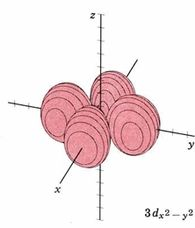A three dimensional axes is shown that are perpendicular to each other. The "x" axes is pointing towards us, the "y" axes is pointing towards the right and the "z" axes is pointing straight upwards. There are four three-dimensional structures that are located on the "x" "y" plane. The structures are lobe shaped and are located on each side of the axial lines.Gif of three dimensional structure rotating about the vertical "z" axes. The structure lies on the "x" and "y" plane and consist of four lobes forming a "X" shape centralized at the origin. The top half surface of the four lobes make up a continuous spectrum of colors from red to yellow to green to blue and to purple. The purple regions make up the bottom half of the lobes and makes a major portion of the structure.
3dyz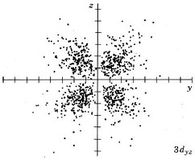A vertical and horizontal axes is labeled "z" and "y" respectively. There is a lobe shaped region of concentrated black dots in each quadrant of the axis which collectively makes an "X" shaped area centralized on the axis origin. There are fewer and fewer black dots as we move away from the axis origin.A three dimensional axes is shown that are perpendicular to each other. The "x" axes is pointing towards us, the "y" axes is pointing towards the right and the "z" axes is pointing straight upwards. There are four three-dimensional structures that are located on each quadrant of the "z" "y" plane. The structures are lobe shaped and form a "X" shape about the origin.Gif of a three dimensional structure rotating about the vertical "z" axes. The structure lies on the "x" and "z" plane and consist of four lobes forming a "X" shape centralized at the origin. The surface of the lobes have a continuous spectrum of color from red to yellow to green, blue and purple. The spectrum of color moves around the surface of each lobe according to its rotating position. The red, yellow, green and blue region moves around the surface of the lobes to always accumulate on the right side of the structure. The purple region make up the rest of the structure as it rotates.

The same pattern extends to n = 4 where four orbital types, namely, 4s, 4p, 4d and 4f, are found. While none of these orbitals will be shown, the patterns seen in moving from 1s to 2s or from 2p to 3p continue with the s, p, and d orbitals. The new f orbitals are even more complicated than the d orbitals. For an understanding of general chemistry, it is important to know that there are seven different orientations for f orbitals, since the number of orbitals of each type (s, p, d, etc.) is important in determining the shell structure of the atom.

1 All dot density diagrams and boundary-surface diagrams are Copyright © 1975 by W. G. Davies and J. W. Moore.

2 The letters s, p, d and f originate from the words sharp, principal, diffuse and fundamental which were used to describe certain features of spectra before wave mechanics was developed. They later became identified with orbital shapes.

This page titled 5.8: Orbitals is shared under a CC BY-NC-SA 4.0 license and was authored, remixed, and/or curated by Ed Vitz, John W. Moore, Justin Shorb, Xavier Prat-Resina, Tim Wendorff, & Adam Hahn.